# Inventory Management – Financial Management MCQ

Inventory Management – CS Executive Financial and Strategic Management MCQ Questions with Answers you can quickly revise the concepts.

## Inventory Management – Financial Management MCQ

Question 1.
Raw materials are directly identifiable as part of the final product and are classified as
(A) Period costs
(B) Fixed costs
(C) Direct materials
(D) Any of the above
(C) Direct materials

Question 2.
Inventory consists of
(A) Intangible property
(B) Tangible property
(C) (A) or (B)
(D) (A) & (B)
(B) Tangible property

Question 3.
Which of the following statement is correct in relation to “need for proper inventory control”?
(B) Materials do not constitute a significant part of the total production cost hence proper planning and controlling of inventories is not a big deal.
(C) Funds are not tied up in surplus stores and stocks.
(D) All of the above

Question 4.
Inventory is valued at ………….
(A) Replacement price
(B) Replacement price or purchase value, whichever is less.
(C) At cost or net realizable value which-ever is less.
(D) Replacement price or net realizable value, whichever is less.
(C) At cost or net realizable value which-ever is less.

Question 5.
………… indicates the level of each particular item of stock at any point of time.
(A) Bill of Material
(B) Material Requisition Note
(C) A bin card
(D) All of the above
(C) A bin card

Question 6.
Which of the following details are recorded in bin card?
(A) Date of order and suppliers name along with address
(B) Record of quantities only
(C) Record of both quantities & values
(D) All of the above
(B) Record of quantities only

Question 7.
Inventory held for sale in the ordinary course of business is known as ………….
(A) Finished Goods
(B) Raw Material
(C) Work-in-progress
(D) Miscellaneous inventory
(A) Finished Goods

Question 8.
…………… is a list of materials, with specifications, material codes and quantity of each material required for a particular job, process or production unit.
(A) Material Transfer Note
(B) Bill of Materials
(C) Purchase Requisition
(D) Bin Card
(B) Bill of Materials

Question 9.
Which of the following method is based on the assumption that, latest consignment of materials or goods manufactured are exhausted first and the closing stock is valued at the cost of earliest lot in hand?
(A) FIFO Method
(B) Highest-in-first-out method
(C) Average cost method
(D) LIFO Method
(A) FIFO Method

Question 10.
…………….. are those cost, which can be identified and traceable to particular product or costing unit or cost centre.
(A) Indirect material costs
(B) Period costs
(C) Direct material costs
(D) Fixed costs
(C) Direct material costs

Question 11.
Wood used in production of tables and chairs, steel bars used in steel factory etc are the examples of …………
(A) Indirect material
(B) Direct material
(C) Fixed material
(D) All of the above
(B) Direct material

Question 12.
Under of inventory valuation, the historical cost of inventory is estimated by calculating at selling price and then deducting an amount equal to the estimated gross margin of profit on such stocks.
(A) Simple Average Price Method
(B) Weighted Average Price Method
(D) Market Price Method

Question 13.
When materials are unloaded, the warehouse staff checks the material unloaded with the delivery note. Then the warehouse staff prepares a…………, a copy of which is given to the supplier’ carrier as a proof of delivery.
(A) Delivery note
(B) Material receipt note
(C) Bill of Material
(D) Purchase Requisition
(B) Material receipt note

Question 14.
……….. .. are those items, which are moving at a slow rate and this may arise due to general depression in demand due to keen g competition.
(A) Dormant stocks
(B) Written-off stocks
(C) Slow moving stocks
(D) Any of the above
(C) Slow moving stocks

Question 15.
CIMA defines ………… as, an internal instruction to a buying office to procure goods or services.
(A) Bin card
(B) Store accounting
(C) Bill of Material
(D) Purchase requisition
(D) Purchase requisition

Question 16.
In which of the following posting is done before the transaction takes place?
(A) Bill of Material
(B) Bin card
(C) Purchase requisition
(D) General ledger
(B) Bin card

Question 17.
……………….. are those items which are not moving temporarily but their movement is expected shortly.
(A) Slow moving stock
(B) Dormant stocks
(C) Non marketable stock
(D) Less efficient stock
(B) Dormant stocks

Question 18.
When materials are delivered, a supplier’s carrier will usually provide a document called to confirm the details of delivery.
(A) Material Transfer Note
(B) Materials Inspection Note
(C) Delivery Note
(D) Purchase Requisition
(C) Delivery Note

Question 19.
………… represents the unusable loss,which can be sold. It is a residue, which is measurable and has a minor value.
(A) Waste
(B) Scrap
(C) Spoilage
(D) Defective
(B) Scrap

Question 20.
If small quantities of direct material used in the end product like gums and threads are used in binding books then it may be categorized as
(A) Miscellaneous cost
(B) Preliminary cost
(C) Indirect material cost
(D) Fixed cost of production
(C) Indirect material cost

Question 21.
Under which of the following method of inventory valuation, the closing inventory is valued at the lowest possible price?
(A) Standard cost method
(C) Specific identification method
(D) Highest-in-first-out method
(D) Highest-in-first-out method

Question 22.
Bill of material acts as an authorization to the in procuring the materials and the concerned department in material requisition from the stores.
(A) Manufacturing department
(B) Store department
(C) Research department
(D) Sales department
(B) Store department

Question 23.
………. records the quantity details,rates and values of stock movements.
(A) Stores ledger
(B) Sales ledger
(C) Material Transfer Note
(D) Delivery Note
(A) Stores ledger

Question 24.
…………… are that portion of the process loss, which can be converted into a finished product by incurring more material and labour expenses.
(A) Waste
(B) Scrap
(C) Spoilage
(D) Defectives
(B) Scrap

Question 25.
CIMA defines as, ……….. “the recording as they occur of receipts, issues and the resulting balances of individual items of stock in either quantity or quality and value”.
(A) Pre-paid Inventory System
(B) Continuous Stock Taking
(C) Perpetual Inventory System
(D) Budgetary Control System
(C) Perpetual Inventory System

Question 26.
Which of the following accounting treatment is correct in relation to “Spoilage”?
(A) Loss due to spoilage can be debited to the job /product /process in which it occurred.
(B) It may be charged to factory over-heads so that the loss is borne by all products.
(C) If spoilage occurs on a specific job/ special order, it is charged to that job itself.
(D) All of the above
(D) All of the above

Question 27.
Which of the following technique can be used for inventory control?
(A) Standard Costing
(B) ABC Analysis
(C) Integrated Accounting System
(D) Any of the above
(B) ABC Analysis

Question 28.
…………….. is an optimum quantity of material to be ordered every time an order is placed. EOQ may be defined as that quantity of purchase which minimizes material order cost and material carrying cost.
(A) Quantity in such lot which has maximum discount
(B) Special Order Quantity (SOQ)
(C) Standard Order Quantity (SOQ)
(D) Economic Order Quantity (EOQ)
(D) Economic Order Quantity (EOQ)

Question 29.
The model and formula of EOQ was developed by in 1913.
(A) F.W. Taylor
(B) F. Wilson Harris
(C) F. Walter Harris
(D) F.W. Marshall
(B) F. Wilson Harris

Question 30.
………. are those materials or components which are so damaged in the manufacturing process that they cannot be repaired or reconditioned.
(A) Spoilage
(B) Waste
(C) Scrap
(D) Defective
(A) Spoilage

Question 31.
Under which of the following plan the analyst lays down a minimum and maximum quantity for each stock item keeping in view its usage, requirements and margin of safety required to minimize risk of stock outs?
(A) ABC plan
(B) Two-bin system
(C) Order cycling systems
(D) Min-Max plan
(D) Min-Max plan

Question 32.
Under …………. a pre-determined price is fixed for valuing each material.
(A) Base Stock Method
(B) Specific Identification Method
(C) Market Price Method
(D) Standard Cost Method
(D) Standard Cost Method

Question 33.
………….. may be defined as that quantity of purchase which minimizes material order cost and material carrying cost.
(A) Basic Ordering Quantity
(B) Constant Ordering Quantity
(C) Economic Order Quantity
(D) Any of the above
(C) Economic Order Quantity

Question 34.
Which of the following statement is true in relation to ABC Analysis of inventory control?
(A) Category A: It contains a relatively large number of inexpensive items.
Category B: It contains inventory items, which are neither very expensive nor very cheap.
Category C: It contains inventory items, which are in massive quantities.
(B) Category A: It contains inventory items, which are neither very expensive nor very cheap. Moreover, they are used in moderate quantities.
Category B: It contains a relatively large number of items. But they are either very inexpensive items or used in very small quantities so that they do not constitute small percentage of the total value of inventories.
Category C: It contains inventory items, which are expensive or used in massive quantities. Thus, they low in quantity but high in value.
(C) Category A: It contains inventory items, which are low in quantity but high in value.
Category B: It contains inventory items, which are neither very expensive nor very cheap. They are used in moderate quantities.
Category C: It contains inventory items, which are in massive quantities, but they are very inexpensive
(D) Any of the above
(C)

Question 35.
Which of the following is property of “Normal Waste”?
(A) It is included in output quantity.
(B) It do not involves further costs of disposing
(C) It is avoidable and controllable
(D) None of the above
(D) None of the above

Question 36.
…………….. are goods/units which can be converted into a finished product by incurring more material & labour expenses.
(A) Scrap
(B) Waste
(C) Spoilage
(D) Defectives
(D) Defectives

Question 37.
Under ………… a continuous record of receipt and issue of materials is maintained by the stores department and the information about the stock of material is always available.
(A) Perpetual Inventory System
(B) Continuous Stock Taking
(C) Periodic Inventory System
(D) Just in time
(A) Perpetual Inventory System

Question 38.
Reorder Level = Safety Stock + …………..
(A) Maximum re-order period
(B) Maximum usage
(C) Minimum consumption

Question 39.
Which of the following treatment is correct for “Waste”?
(A) Abnormal waste is unavoidable and uncontrollable and treated as part of the product cost.
(B) Normal waste is transferred to the Costing P & L A/c
(C) Both (a) & (b)
(D) Neither (a) nor (b)
(D) Neither (a) nor (b)

Question 40.
Which of the following is required in order to calculate EOQ?
(A) Cost of equity (Ke)
(B) Stock-out Cost
(C) Opportunity Cost
(D) All of the above
(B) Stock-out Cost

Question 41.
Which of the following formula is used to calculate Re-order Level?
(A) (Maximum usage × Maximum re-order period)
(B) Safety Stock + Normal lead time consumption
(C) (Average usage × Average re-order period)
(D) (A) or (B)
(D) (A) or (B)

Question 42.
Which of the following is/are example of “Waste” in relation to material cost?
(A) Smoke
(B) Sawdust in timber industry
(C) Portion of the process loss, which can be converted into a finished product
(D) All of the above
(A) Smoke

Question 43.
Which of the following formula is used to calculate Maximum Level?
(A) (Re-order level + Re-order qty) – (Maximum consumption × Maximum reorder period)
(B) (Re-order level + Re-order qty) – (Minimum consumption × Minimum reorder period)
(C) (Re-order level 4- EOQ) – (Minimum consumption × Minimum re-order period)
(D) (B) or (C)
(D) (B) or (C)

Question 44.
Re-order Level is also known as ……………
(A) Re-order Quantity
(B) Economic order quantity
(C) Reorder point
(D) (A) or (C)
(D) (A) or (C)

Question 45.
Danger Level = ?
(A) (Maximum consumption × Lead time for emergency purchase
(B) (Average consumption × Lead time for emergency purchase)
(C) (Minimum × Lead time for emergency purchase
(D) Ordering Level – (Average Usage × Re-order Period)
(B) (Average consumption × Lead time for emergency purchase)

Question 46.
………… purchase means the purchase of goods or material such that delivery immediately precedes their use.
(A) Economic order quantity
(B) Reorder point
(C) Re-order Quantity
(D) Just in time (JIT)
(D) Just in time (JIT)

Question 47.
(Maximum usage – Average Usage) × Lead Time = ?
(A) Re-order Point
(B) Danger Level
(C) Safety Stock Level
(D) Reorder Level
(C) Safety Stock Level

Question 48.
Which of these is not a Material control technique:
(A) ABC Analysis
(B) Fixation of raw material levels
(C) Maintaining stores ledger
(D) Control over slow moving and non moving items
(C) Maintaining stores ledger

Question 49.
Out of the following, what is not the work of purchase department?
(A) Receiving purchase requisition
(B) Exploring the sources of material supply
(C) Preparation and execution of purchase orders

Question 50.
Bin Card is a ……………….
(A) Quantitative as well as value wise records of material received, issued and balance
(B) Quantitative record of material received, issued and balance
(C) Value wise records of material received, issued and balance
(D) A record of labour attendance
(B) Quantitative record of material received, issued and balance

Question 51.
Stores Ledger is a:
(A) Quantitative as well as value wise records of material received, issued and balance
(B) Quantitative record of material received, issued and balance
(C) Value wise records of material received, issued and balance
(D) A record of labour attendance
(A) Quantitative as well as value wise records of material received, issued and balance

Question 52.
Economic order quantity is that quantity at which cost of holding and carrying inventory is
(A) Maximum and equal
(B) Minimum and equal
(C) It can be maximum or minimum depending upon case to case
(D) Minimum and unequal
(B) Minimum and equal

Question 53.
ABC analysis is an inventory control technique in which:
(A) Inventory levels are maintained
(B) Inventory is classified into A, B and C category with A being the highest quantity, lowest value.
(C) Inventory is classified into A, B and C Category with A being the lowest quantity, highest value
(D) Either (B) or (C)
(C) Inventory is classified into A, B and C Category with A being the lowest quantity, highest value

Question 54.
Which one out of the following is not an inventory valuation method?
(A) FIFO
(B) LIFO
(C) Weighted Average
(D) EOQ
(D) EOQ

Question 55.
In case of rising prices (inflation), FIFO method will:
(A) Provide lowest value of closing stock and profit
(B) Provide high est value of closing stock and profit
(C) Provide highest value of closing stock but lowest value of profit
(D) Provide highest value of profit but lowest value of closing stock
(B) Provide high est value of closing stock and profit

Question 56.
In case of rising prices (inflation), LIFO method will:
(A) Provide lowest value of closing stock and profit
(B) Provide highest value of closing stock and profit
(C) Provide highest value of closing stock but lowest value of profit
(D) Provide highest value of profit but lowest value of closing stock
(A) Provide lowest value of closing stock and profit

Question 57.
Cost of abnormal wastage is:
(A) Charged to the product cost
(B) Charged to the profit & loss account
(C) Charged partly to the product and partly profit & loss account
(D) Not charged at all.
(B) Charged to the profit & loss account

Question 58.
The average annual consumption of material is 20,000 kg at a price of ₹ 2 per kg. The storage cost is 16% on average inventory and the cost of placing one order is ₹ 50. How much is to be purchased at a time?
(A) 2,500 kg
(B) 2,000 kg
(C) 2,532 kg
(D) 2,352 kg
(A) 2,500 kg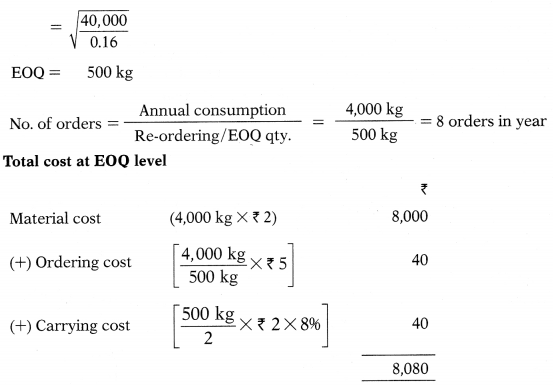Question 59.
Annual consumption of material – 4,000 units
Ordering Cost – ₹ 5
Cost per unit – ₹ 2
Storage & carrying cost – 8% p.a.
Economic Order Quantity for the item is:
(A) 500 units
(B) 800 units
(C) 300 units
(D) 400 units
(A) 500 units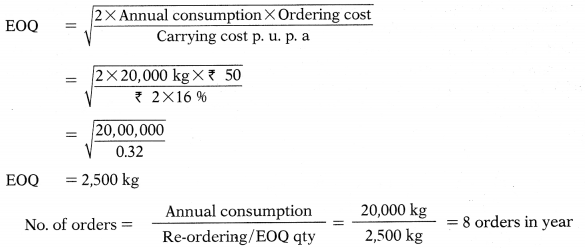Question 60.
The annual demand of a certain component bought from the market is 1,000 units. The cost of placing an order is ₹ 60 and the carrying cost per unit is ₹ 3 p.a. The Economic Order Quantity for the item is ?..
(A) 200 units
(B) 400 units
(C) 600 units
(D) 500 units
(A) 200 units
$$\mathrm{EOQ}=\sqrt{\frac{2 \times 1,000 \text { units } \times \text { Rs. } 60}{\text { Rs. } 3}}$$
= 200

Question 61.
For a particular item of store, the following information are available:
Re-order quantity =12 units
Maximum consumption per week = 300 units
Normal consumption per week = 200 units Re-order period = 2 to 4 weeks The Re-order level = ?
(A) 600 units
(B) 400 units
(C) 1,200 units
(D) None of the above
(C) 1,200 units
Re-order level (Maximum usage X Maximum delivery period)
= (300 units × 4 weeks)
1,200 units

Question 62.
Which of the following items can be classified as “A” as per ABC Analysis of inventory control?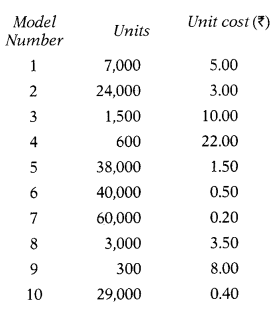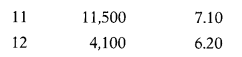(B)

Question 63.
Which of the following items can be classified as “C” as per ABC Analysis of inventory control?(A) Item number 5 only
(B) Item number 5, 4
(C) Item number 3, 4
(D) Item number 5, 2
(A) Item number 5 only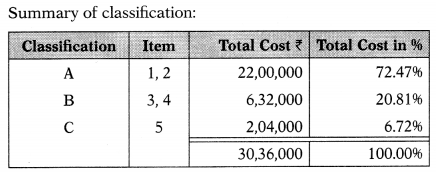Question 64.
A manufacturer requires 9,600 units of a certain component annually. This is currently purchased from a regular supplier at ₹ 50 per unit. The cost of placing an order is ₹ 60 per order and the annual carrying cost is ₹ 5 per price. Annual ordering plus carrying cost = ?
(A) 2,400
(B) 480
(C) 4,800
(D) 240
(A) 2,400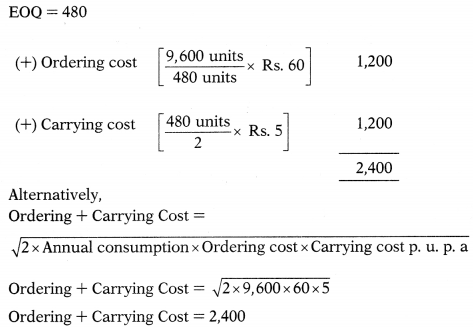Question 65.
A publishing house purchases 2,000 units of a particular item per year at a unit cost of ₹ 20. The ordering cost per order is ₹ 50 and the inventory carrying cost is 25%. How will be the total cost if company decides to buy in EOQ?
(A) 41,325
(B) 41,000
(C) 41,500
(D) 41,525
(B) 41,000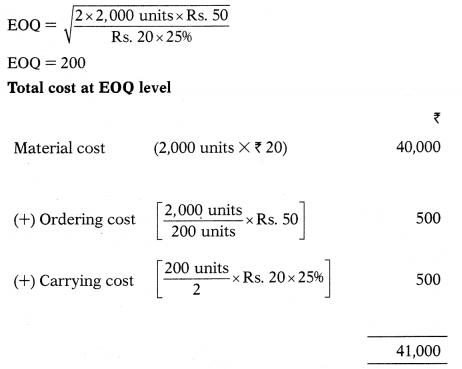Question 66.
A factory requires 1,500 units of an item per month. The cost of each unit is 27. The cost per order is 150 and inventory carrying charge works Out to 20% of average inventory. Supplier offers 2% price discount on a minimum supply of 1,200 units. How much money will be saved h accepting suppliers offer?
(A) 6,995
(B) 9,695
(C) 12,870
(D) Nothing will be saved and company will have to incur extra cost.
(B) 9,695Question 67.
JP Ltd., manufactures of a special product, follows the policy of EOQ for one of its components. The component’s details are as follows:
Purchase price per component: ₹ 200
Cost of an order: ₹ 100
Annual cost of carrying one unit in inventory: 10% of purchase price
Total cost of inventory and ordering per annum: ₹ 4,000
Compute the EOQ.
(A) 200 units
(B) 400 units
(C) 600 units
(D) 800 units
(A) 200 units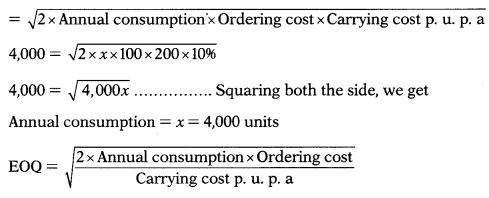Question 68.
A firm requires 16,000 units of a certain component which it buys at ₹ 60 each. The cost of placing an order and following it up is ₹ 120 and the annual storage charges works out to 10% of the cost of the item. To get maximum benefit the firm should place order for at a time.
(A) 1,000 units
(B) 900 units
(C) 800 units
(D) 700 units
(C) 800 units
EOQ = 800 units

Question 69.
The average annual consumption of material is 20,000 kg at a price of ₹ 2 per kg. The storage cost is 16% on average inventory and the cost of placing one order is ₹ 50. What is the time gap between two orders?
(A) 7 orders in year
(B) 8 orders in year
(C) 9 orders in year
(D) 6 orders in year
(B) 8 orders in year
EOQ = 2,500Question 70.
G Ltd. produces a product, which has a monthly demand of 4,000 units. The product requires a component X, which is purchased at ₹ 20. For every finished product, one unit of component is required. The ordering cost is ₹ 120 per order and the holding cost is 10% p.a. EOQ = ?
(A) 2,400 units
(B) 4,200 units
(C) 4,400 units
(D) 2,200 units
(A) 2,400 units
Annual consumption = 4.000 × 12 = 48,000
$$\mathrm{EOQ}=\sqrt{\frac{2 \times 48,000 \text { units } \times \text { Rs. } 120}{\text { Rs. } 20 \times 10 \%}}$$
= 2,400 units

Question 71.
In a Company the weekly minimum and maximum consumption of Material-A are 25 and 75 emits respectively. The re-order quantity as fixed by the company is 300 units. Material-A is received within 4 to 6 weeks from the date of supply order.
Minimum Level = ?
(A) 450 units
(B) 200 units
(C) 650 units
(D) 800 units
(B) 200 units
Re-order = (Maximum usage × Maximum delivery period) level
= (75 units × 6 weeks)
= 450 units
Minimum = Re-order level – (Average usage × Average delivery period) level
= 450 units – (50 units × 5 weeks)
= 200 units

Question 72.
A company manufactures several components in batches. The following data relates to one component:
Annual demand: 32,000 units;
Set-up cost per batch: ₹ 120.
Annual rate of interest: 12%;
Cost of production per unit: ₹ 16.
The Economic Batch Quantity is
(A) 2,500 units
(B) 4,000 units
(C) 3,000 units
(D) 2,000 units
(D) 2,000 unitsQuestion 73.
G Ltd. produces a product, which has a monthly demand of 4,000 units. The product requires a component X, which is purchased at ₹ 20. For every finished product, one unit of component is required. The ordering cost is ₹ 120 per order and the holding cost is 10% p.a. At EOQ level purchase,Ordering Cost + Carrying Cost = ?
(A) 2,400
(B) 4,800
(C) 2,800
(D) 4,400
(B) 4,800
Annual consumption = 4,000 × 12 48,000
Ordering + Carrying CostQuestion 74.
Raw material price =₹ 60 per kg., Handling cost = ₹ 360, Freight = ₹ 390 per order, Incremental carrying cost of inventory of raw material = ₹ 0.50 per kg per month. Cost of working capital finance on the investment in inventory = ₹ 9 per kg p.a. Annual production = 1,00,000 units.
2.5 units are obtained from one kg of raw material. EOQ = ?
(A) 2,000 kg
(B) 3,000 kg
(C) 4,000 kg
(D) 5,000 kg
(A) 2,000 kg
Calculation of annual consumption:
For 2.5 finished unit – 1kg of raw material required
For 1,00,000 units -?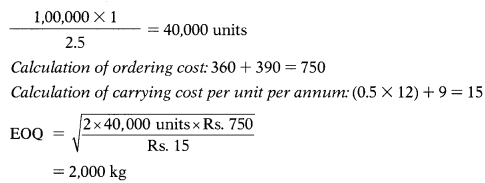Question 75.
If the minimum stock level and average stock level of raw material A are 4,000 and 9,000 units respectively, find out its “Re-order quantity”.
(A) 10,000 units
(B) 5,000 units
(C) 2,500 units
(D) 26,000 units
(A) 10,000 units
Let the Re-order quantity be ‘x’.
Average Stock Level Minimum Stock Level + Re-order quantity
9,000 = 4,000 + ½ x
X = 10,000 units

Question 76.
Re-order quantity of material X is 5,000 kg.; Maximum level 8,000 kg.; Minimum usage 50 kg. per hour; minimum re-order period 4 days; daily working hours in the factory is 8 hours. You are required to calculate the re-order level of material X.
(A) 4,600 kg
(B) 400 kg
(C) 4,200 kg
(D) 11,400 kg
(B) 400 kg
Let the Re-order Level be ‘x
Maximum level = (Re-order level + Re-ordering qty) – (Minimum usage X
Minimum delivery period)
8,000 (x units + 5,000 kg) – (400 kg X 4 days)
8,000 = x-3,400 kg
x = 4,600kg
Minimum usage = 50 kg × 8 hours 400 kg

Question 77.
A manufacturer used 400 units of a Component every month and buys them entirely from an outside supplier @ ₹ 40 per unit. The order placing and receiving cost is ₹ 100 and storage and carrying cost is 15% of the value of Stock. To get maximum benefit the manufacturer should place order at a time for ………
(A) 300 units
(B) 400 units
(C) 450 units
(D) 500 units
(B) 400 units
$$\mathrm{EOQ}=\sqrt{\frac{2 \times 4,800 \text { units } \times \text { Rs. } 100}{40 \times 15 \%}}$$
= 400 units

Question 78.
Normally delivery takes place in 6 days. 1-day stock will be safety stock. Average consumption per day 150 units.
Re-order Point = ?
(A) 1,050 units
(B) 1,350 units
(C) 900 units
(D) None of the above
(A) 1,050 units
Re-order Point = 7 × 150 = 1,050 units
Alternatively, (6 × 150) + (1 × 150) = 900 + 150 = 1,050

Question 79.
Minimum Level = 2,750 units, Re-order level = 6,000 units, Average delivery period = 6.5 weeks. Normal usage = ?
(A) 500 units
(B) 750 units
(C) 400 units
(D) 350 units
(A) 500 units
Let the normal usage be ‘X’
Minimum Level Re-order level – (Normal usage × Average delivery period)
2,750 units 6,000 units – (x X 6.5 weeks)
Normal usage 500 units
Let the normal usage be ‘X’
Minimum Level Re-order level – (Normal usage × Average delivery period)
2,750 units 6,000 units – (x × 6.5 weeks)
Normal usage 500 units

Question 80.
Maximum Level = 6,832 units Re-order level = 6,000 units Minimum usage = 250 units Minimum delivery period = 5 weeks. EOQ/Re-order quantity = ?
(A) 2,082 units
(B) 4,791 units
(C) 3,791 units
(D) 2,750 units
(A) 2,082 units
Let the Re-ordering qty be ‘x’
Maximum Level = (Re-order level + Re-ordering qty) – (Minimum usage X Minimum delivery period)
6,832 units = (6,000 units + x) – (250 units X 5 weeks)
Re-ordering qty = 2,082

Question 81.
PQR Ltd. produces a product, which has a monthly demand of 52,000 units. The product requires a Component X, which is purchased at ₹ 15 per unit. For every finished product, 2 units of component X are required. The ordering cost is ₹ 350 per order and the carrying cost is 12% p.a. If the minimum lot size to be supplied is 52,000 units. What is the extra cost, the company has to incur?
(A) 19,827
(B) 15,545
(C) 16,827
(D) 19,828
Answer next 5 question on the basis of(B)

Question 82.
Re-order quantity = ?
(A) 115.47 units
(B) 186.19 units
(C) 188.47 units
(D) 166 units
(B) 186.19 units

Question 83.
Re-order level = ?
(A) 368 units
(B) 536 units
(C) 200 units
(D) 450 units
(D) 450 units

Question 84.
Minimum level = ?
(A) 368 units
(B) 536 units
(C) 200 units
(D) 450 units
(C) 200 units

Question 85.
Maximum level = ?
(A) 368 units
(B) 536 units
(C) 200 units
(D) 450 units
(B) 536 units

Question 86.
Average stock level = ?
(A) 368 units
(B) 536 units
(C) 200 units
(D) 450 units
(A) 368 units

Question 87.
Pooja Pipes Ltd. uses about 75,000 valves per year and the usage is fairly constant at 6,250 valves per month. The valve costs ₹ 1.50 per unit when bought in large quantities; and the carrying cost is estimated to be 20% of average inventory investment on an annual basis. The cost to place an order and process the delivery is ₹ 18. Frequency of order = ?
(A) 25 orders per year
(B) 52 orders per year
(C) 20 orders per year
(D) 50 orders per year
(A) 25 orders per year
$$\text { EOQ }=\sqrt{\frac{2 \times 75,000 \text { units } \times \text { Rs. } 18}{1.5 \times 20 \%}}$$
= 3,000 unitsQuestion 88.
Average – 10 days
Maximum – 15 days
Minimum – 6 days
Emergency purchases – 4 days.
Danger level = ?
(A) 440 units
(B) 150 units
(C) 440 units
(D) 60 units
(D) 60 units
Danger level = (Average consumption × Lead time for emergency purchase)
= (15 × 4 days)
= 60 units

Question 89.
Average monthly market demand = 2,000 tubes, Ordering cost = ₹ 100 per order, Inventory carrying cost = 20% per annum, Cost of tubes = ₹ 500 per tube, Normal usage =100 tubes per week. If the supplier is willing to supply quarterly 1,500 units at a discount of 5%, is it worth accepting?
(A) EOQ purchase is the best policy of purchase and hence there is no need to accept any discount offer form supplier.
(B) Do not accept offer of quarterly supply of 1,500 tubes at 5% discount as it will increase total annual cost.
(C) Accept offer of quarterly supply of 1,500 tubes at 5% discount as it will save ₹ 64,851.
(D) Accept offer of quarterly supply of 1,500 tubes at 5% discount as it will save ₹ 68,601.
(D) Accept offer of quarterly supply of 1,500 tubes at 5% discount as it will save ₹ 68,601.
Annual consumption = Normal usage × No. weeks in year
= 100 tubes × 52 weeks
= 5,200 tubes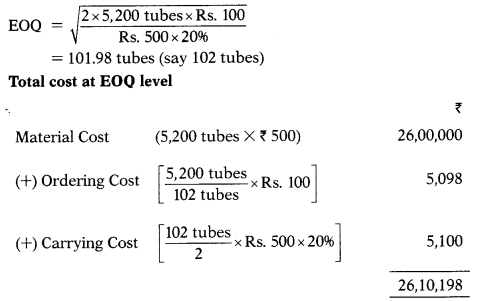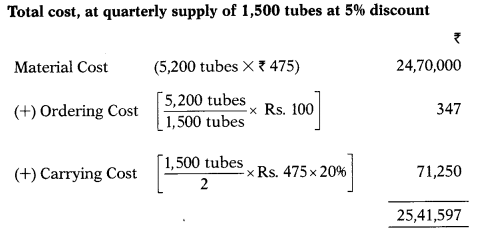Question 90.
About 50 items are required every day for a machine. A fixed cost of ₹ 50 per order is incurred for placing an order. The inventory carrying cost per item amount to ₹ 0.02 per day. The lead period is 32 days. Re-order Level = ?
(A) 500 units
(B) 1,600 units
(C) 1,100 units
(D) 600 units
Answer next 5 question on the basis of following data:(B) 1,600 units

Question 92.
Re-order level = ?
(A) 189.5 units
(B) 207 units
(C) 92 units
(D) 287 units
(B) 207 units

Question 93.
Minimum level = ?
(A) 189.5 units
(B) 207 units
(C) 92 units
(D) 287 units
(C) 92 units

Question 94.
Maximum level = ?
(A) 92 units
(B) 189.5 units
(C) 207 units
(D) 287 units
(D) 287 units

Question 95.
Average stock level = ?
(A) 287 units
(B) 92 units
(C) 189.5 units
(D) 207 units
(C) 189.5 units

Question 96.
A Company manufactures 5,000 units of a product per month. The cost of placing an order is ₹ 100. The purchase price of the raw material is ₹ 10 per kg. The reorder period is 4 to 8 weeks. The consumption of raw material varies from 100 kg. to 450 kg per week, the average consumption being 275 kg. The carrying cost of inventory is 20% p.a. EOQ = ?
(A) 14,300 kg
(B) 1,196 kg
(C) 4,396 kg
(D) 3,173 kg
(B) 1,196 kg
Annual consumption = Average consumption × No. weeks in year
=275kg × 52
= 14,300kg

Question 97.
Re-order Level = 3,750 units, Minimum Level = 1,750 units, Average Delivery Period=2 weeks, Average Consumption=?
(A) 1,750 units
(B) 1,250 units
(C) 1,500 units
(D) 1,000 units
(D) 1,000 units
Minimum Level = Re-order Level – (Average Consumption × Average Delivery Period)
1,750 units = 3,750 units -( x × 2 weeks)
x = Average Consumption = 1,000 units

Question 98.
Normally delivery takes place in 6 days. 3 day stock will be safety stock. Average consumption per day 150 units. Minimum consumption per day is 75 units. Re-order Point ?
(A) 1,125 units
(B) 675 units
(C) 900 units
(D) 1,350 units
(D) 1,350 units
Re-order Point = 9 × 150 = 1,350 units
Alternatively, (6 × 150) + (3 × 150) – 900 + 450 = 1,350

Question 99.
Annual consumption = 54,000 castings, EOQ = 300 castings, Time gap between two orders = ?
(A) 2 days
(B) 180 days
(C) 0.5 days
(D) 90 days
(A) 2 days

Question 100.
Safety Stock = 30 days consumption,
Annual consumption (360 days) = 12,000 units, Normal lead time =15 days. Re- g order Level = ?
(A) 1,096 units
(B) 1,500 units
(C) 1,000 units
(D) 500 units
(B) 1,500 units
Normal Lead time consumption = $$\frac{12,000}{360}$$ × 15 = 500 units
Re-order Level = (Safety Stock + Normal lead time consumption) = (1,000 units + 500 units)
= 1,500 units

Question 101.
Minimum Level = 2,750 units, EOQ = 2,082 units, Average Level = ?
(A) 4,832 units
(B) 3,457 units
(C) 3,791 units
(D) 4,791 units
(C) 3,791 units
Average Level = Minimum Level + b Re-ordering Quantity
= 2,750 units + 1/2 2,082 units
= 3,791 units

Question 102.
Time gap between two orders = 3.6 days, Ordering Quantity = 400 packs,
No. of days in a year = 360 days, Annual Consumption = ?
(A) 41,111 packs
(B) 40,000 packs
(C) 44,444 packs
(D) 55,555 packs
(B) 40,000 packsQuestion 103.
The annual carrying cost of material ‘X’ is ₹ 3.6 per unit and its total carrying cost is ₹ 9,000 per annum. What would be the economic order quantity for material ‘X’, if there is no safety stock of material X?
(A) 5,000 units
(B) 16,200 units
(C) 682 units
(D) None of the above
(A) 5,000 units
Carrying Cost = $$\frac{\mathrm{EOQ}}{2}$$ Carrying cost p. u. p. a
9,000 = $$\frac{\mathrm{EOQ}}{2} \times 3.6$$
EOQ = 5,000 Units.

Question 104.
Opening Stock = 25,000 units, Closing Stock = 15,000 units, Purchases = 1,90,000 units. (Take 1 year = 360 days). Stock Velocity = ?
(A) 30 days
(B) 90 days
(C) 60 days
(D) 36 days
(D) 36 daysQuestion 105.
Inventory turnover ratio = 2.5 times, Opening Stock=90,000 units, Closing Stock = 1,10,000 units. Purchases = ?
(A) 2,30,000 units
(B) 2,70,000 units
(C) 2,50,000 units
(D) 2,40,000 units
(B) 2,70,000 units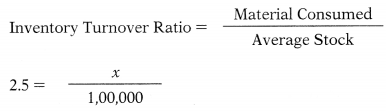Material Consumed = 2,50,000
Opening Stock + Purchases – Closing Stock = Material Consumed
90,000 + Purchases – 1,10,000 = 2,50,000
Purchases = 2,70,000

Question 106.
Stock Velocity =180 days, Material consumed = 1,50,000, Closing Stock = 62,500 units. Opening stock = ?
(A) 2,12,500 units
(B) 3,00,000 units
(C) 87,500 units
(D) 1,62,500 units
(C) 87,500 unitsQuestion 107.
At what price per unit would Part No. A32 be entered in the store Ledger, if following invoice was received from supplier:(A) ₹ 970
(B) ₹ 1,000
(C) ₹ 800
(D) ₹ 850
(D) ₹ 850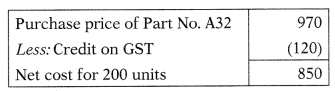Question 108.
EOQ =100 units, Annual consumption = 2,000 units, Carrying cost per unit per annum = ₹ 480, Ordering cost = ?
(A) ₹ 1,400
(B) ₹ 1,200
(C) ₹ 1,600
(D) ₹ 2,000
(B) ₹ 1,200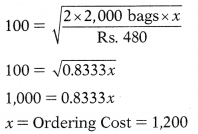Question 109.
No. of deliveries =16 deliveries, EOQ = 80 bags, Carrying cost per unit per annum = ₹ 560, Ordering cost = ₹
(A) ₹ 1,400
(B) ₹ 1,200
(C) ₹1,600
(D) ₹ 2,000
(A) ₹ 1,400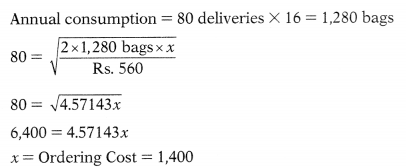Question 110.
Total cost = 24,30,000, No. of orders = 4 orders, Ordering cost = ₹ 750, Ordering qty = 10,000 units, Carrying cost per unit per annum = ₹ 15. Material price per unit =
(A) 58.80
(B) 60.20
(C) 60.80
(D) 60.00
(A) 58.80Question 111.
Calculate the value of closing stock from the following according to FIFO method:
1st January, 2014: Opening balance: 50 units @ ₹ 4
Receipts:
5th January, 2014: 100 units @ ₹ 5
12th January, 2014: 200 units @ ₹ 4.50
Issues:
2nd January, 2014: 30 units
18th January, 2014: 150 units
(A) ₹ 765
(B) ₹ 805
(C) ₹ 786
(D) ₹ 700
(A) ₹ 765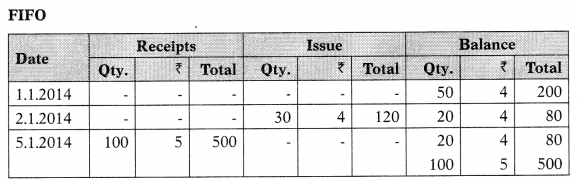Question 112.
Calculate the value of closing stock from the following according to LIFO method:
1st January, 2014: Opening balance: 50 units @ ₹ 4
Receipts:
5th January, 2014:100 units @ ₹ 5
12th January, 2014: 200 units @ ₹ 4.50
Issues:
2nd January, 2014: 30 units
18th January, 2014: 150 units
(A) ₹ 765
(B) ₹ 805
(C) ₹ 786
(D) ₹ 700
(B) ₹ 805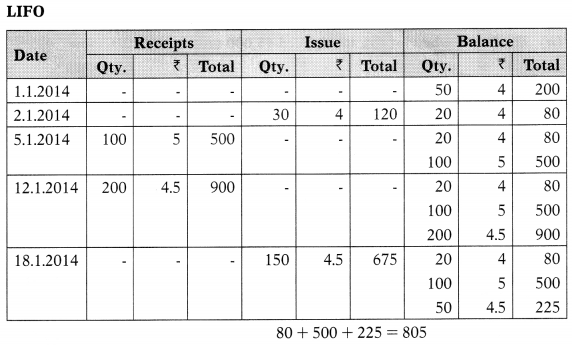Question 113.
Calculate the value of closing stock from the following according to Weighted Average method:
1st January, 2014: Opening balance: 50 units @ ₹ 4
Receipts:
5th January, 2014: 100 units @ ₹ 5
12th January, 2014: 200 units @ ₹ 4.50 Issues:
2nd January, 2014: 30 units
18th January, 2014: 150 units
(A) ₹ 765
(B) ₹ 805
(C) ₹ 786
(D) ₹ 700
(C) ₹ 786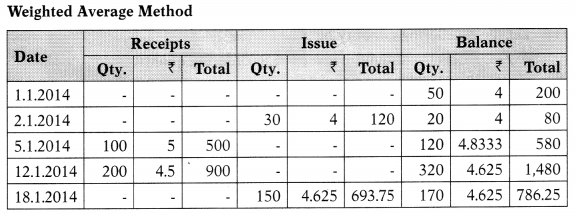Question 114.
Calculate re-order level from the following:
Safety stock: 1,000 units Consumption per week: 500 units
It takes 12 weeks to reach material from the date of ordering.
(A) 1,000 units
(B) 6,000 units
(C) 3,000 units
(D) 7,000 units
(D) 7,000 units
(500 × 12) + 1,000 = 7,000

Question 115.
From the following information, calculate the extra cost of material by following EOQ:
Annual consumption = 45,000 units
Ordering cost per order = ₹ 10
Carrying cost per unit p. a. = ₹ 10
Purchase price per unit = ₹ 50
Re-order quantity at present = 45,000 units
There is discount of 10% per unit in case of purchase of 45,000 units in bulk.
(A) No saving
(B) ₹ 2,00,000
(C) ₹ 2,22,010
(D) ₹ 2,990
(D) ₹ 2,990
EOQ=$$\sqrt{\frac{2 \times 45,000 \text { units } \times \text { Rs. } 10}{\text { Rs. } 10}}$$
= 300 units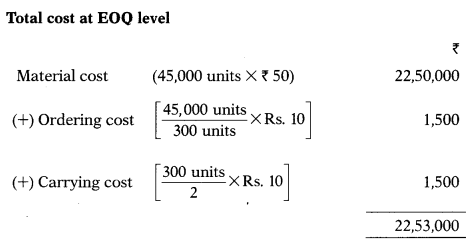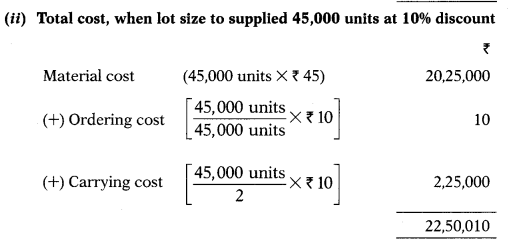Advice: Total cost at suppliers offer is less by 2,990 (22,53,000 – 22,50,010), so suppliers offer is acceptable.

Question 116.
Dec 2014: Which type of material is classified as ‘A’ type in ABC analysis —
(A) High price, more quantity
(B) High price, less quantity
(C) Low price, more quantity
(D) Low price, less quantity
(B) High price, less quantity

Question 117.
Dec 2014: Which of the following formula cannot be used to calculate re-order level —
(A) Minimum level + consumption during lead time
(B) Maximum consumption X maximum re-order period
(C) Maximum consumption X lead time+ safety stock
(D) Minimum consumption X minimum re-order period
(D) Minimum consumption X minimum re-order period

Question 118.
Dec 2014: Which of the following is recorded by bin card —
(A) Quantity
(B) Quantity and value
(C) Value
(D) Quality
(A) Quantity

Question 119.
Dec 2014: If the minimum stock level and average stock level of raw material ‘A’ are 4,000 & 9,000 units respectively, what is its reorder quantity —
(A) 8,000 units
(B) 11,000 units
(C) 10,000 units
(D) 9,000 units
(C) 10,000 units
Let the reordering quantity be ‘x’
Average Level Minimum Level + ½ Reordering quantity
9,000 = 4,000 + 0.5x
5,000 = 0.5x
x = Reordering quantity = 10,000

Question 120.
Dec 2014: The method of regular physical verification of material throughout the year is known as..
(A) Periodic stock taking
(B) Bin card system
(C) Continuous stock taking
(D) Stock ledger system
(C) Continuous stock taking

Question 121.
Dec 2014: In inflationary situation, which system of inventory valuation shows higher profits —
(A) LIFO
(B) FIFO
(C) HIFO
(D) Weighted average
(B) FIFO

Question 122.
Dec 2014: Under which of the following inventory control technique, maximum and minimum level of each stock is laid down —
(A) Min-max plan
(B) Two bin system
(C) Order cycle system
(D) ABC analysis
(A) Min-max plan

Question 123.
Dec 2014: A company manufactures 5,000 units of a product per month. The cost of placing an order is ₹ 100. Purchase price of the raw material is ₹ 10 per kg. Average consumption of raw material is 275 kg per week. The carrying cost of inventory is 20% per annum. The economic order quantity is –
(A) 1,196 kg
(B) 707 kg
(C) 2,449 kg
(D) 2,400 kg
(A) 1,196 kg
Annual consumption = Average consumption X No. weeks in year
275 kg×52
= 14,300kg
$$=\sqrt{\frac{2 \times 14,300 \mathrm{~kg} \times \text { Rs. } 100}{\text { Rs. } 10 \times 20 \%}}$$
EOQ 1,195.83 kg (say 1,196 kg)

Question 124.
June 2015: Under which of the following inventory control techniques, two piles or bundles are maintained for each item of stock —
(A) Min-max plan
(B) Order cycling system
(C) Two-bin system
(D) ABC analysis
(C) Two-bin system

Question 125.
June 2015: A store ledger is a record of receipts, issues and closing balances of material by entering  …………….
(A) Quantity only
(B) Quantity and value
(C) Value only
(D) Quality only
(B) Quantity and value

Question 126.
June 2015: Bill of material acts as an authorization to the stores department in procuring the material and all the materials listed on the bill are sent to the —
(A) Sales department
(B) Production department
(C) Accounts department
(D) Stores department
(B) Production department

Question 127.
June 2015: Which of the following method is based on the assumption that costliest materials are issued first and inventory is valued at the lowest possible price —
(A) FIFO method
(B) UFO method
(C) Highest-in-first-out method
(D) Weighted average method
(C) Highest-in-first-out method

Question 128.
June 2015: For a product-X, following information is available:
Maximum consumption per week: 300 units Normal consumption per week: 200 units Re-order period: 2 to 4 weeks
The re-order level will be —
(A) 400 units
(B) 1,200 units
(C) 600 units
(D) 800 units
(B) 1,200 units
Re-order Level = (Maximum usage × Maximum delivery period)
= (300 units × 4 weeks)
= 1,200 units

Question 129.
June 2015: A company requires 1,500 units, of an item per month. The cost of each unit is ₹ 30. The cost of placing an order is ₹ 200 and the material carrying charges work out to be 20% of the average material. The economic order quantity (EOQ) is —
(A) 1,095 units
(B) 316 units
(C) 490 units
(D) 33 units
(A) 1,095 unitsQuestion 130.
June 2015: Following information is available regarding a product-X:
1st January, 2015:
Opening balance: 50 units @ ₹ 4 Receipts:
5th January, 2015: 100 units @ ₹ 5
12th January, 2015: 200 units @ ₹ 5.50 Issues:
2nd January, 2015: 30 units 18th January, 2015: 170 units
The value of closing stock according to FIFO method is —
(A) ₹ 660
(B) ₹ 770
(C) ₹ 825
(D) ₹ 1,100
(C) ₹ 825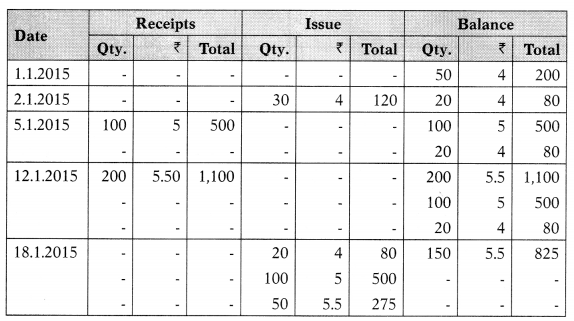Question 131.
June 2015: In case of rising prices, FIFO method will provide –
(A) Lowest value of closing stock and profit
(B) Highest value of closing stock and profit
(C) Highest value of closing stock but lowest value of profit
(D) Lowest value of closing stock but highest value of profit
(B) Highest value of closing stock and profit

Question 132.
Dec 2015: In a company, weekly minimum and maximum consumption of Material-A is 25 and 75 units respectively. The re-order quantity as fixed by the company is 300 units. The material is received within 4 to 6 weeks from issue of supply order. Maximum level of Material-A is —
(A) 640 Units
(B) 650 Units
(C) 175 Units
(D) 560 Units
(B) 650 Units
Reorder Level = (Maximum usage× Maximum re-order period)
= (75 × 6)
= 450
Maximum level = (Re-order level + Re-order qty) – (Minimum consumption × Minimum re-order period)
=(450+300)-(25×4)
= 650

Question 133.
Dec 2015: FIFO method of valuing material issues is suitable in times of —
(A) Rising prices
(B) Falling prices
(C) Price fluctuation
(D) Boom period
(B) Falling prices

Question 134.
Dec 2015: About 50 units are required every day for a machine. Fixed cost of ₹ 50 is incurred for placing an order. The inventory carrying cost per unit amounts to ₹ 0.02 per day. The lead period is 32 days. Economic Order Quantity is —
(A) 200 Units
(B) 300 Units
(C) 500 Units
(D) 100 Units
(C) 500 Units
Annual Consumption = 50 × 365 = 18,250. Carrying cost p. u. p. a = 0.02 × 365 =7.3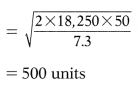Question 135.
June 2016: XYZ Ltd. had 4,000 units of inventory in hand on 1st March, 2016, costing ₹ 4 per unit. Purchases and issues of material during the month were as follows: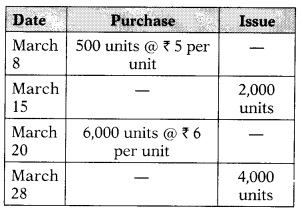The cost of inventory as on 31st March, 2016 under FIFO and weighted average cost method will be
(A) ₹ 27,000 and ₹ 24,498
(B) ₹ 27,000 and ₹ 23,625
(C) ₹ 22,000 and ₹ 23,625
(D) ₹ 22,000 and ₹ 24,498
(A) ₹ 27,000 and ₹ 24,498Question 136.
June 2016: EOQ is 200 units, ordering cost ₹ 20per order and total purchases 4,000 units. The carrying cost per unit will be —
(A) ₹ 2
(B) ₹ 6
(C) ₹4
(D) None of the above
(C) ₹440,000 x 1,60,000
x = 4
Alternatively, students can adopt following procedure to solve the MCQ.
Figure of each option should be put in above formula and check that whether
EOQ comes to 200 or not. If you apply figure of Option (C) ₹ 4 in above formula,
EOQ comes to 200 and hence Option (C) is correct.

Question 137.
June 2016: Which one of the following statements is true in ABC classification of materials —
(A) ‘C’ items of material have moderate % of cost and high % of quantity
(B) ‘A’ items of material have high % of cost and low % of quantity
(C) ‘A’ items of material have high % of cost and high % of quantity
(D) ‘B’ items of material have moderate % of cost and low % of quantity
(B) ‘A’ items of material have high % of cost and low % of quantity

Question 138.
June 2016: In a situation of rising prices, profit and tax liability would be lower under method than under method of material issue pricing.
(A) FIFO; LIFO
(B) LIFO; FIFO
(C) LIFO; Average
(D) FIFO; Average
(B) LIFO; FIFO

Question 139.
June 2016: The technique of economic order quantity is losing significance since the development of —
(A) Perpetual inventory
(B) Just-in-time
(C) First-in-first-out
(D) ABC analysis
(B) Just-in-time

Question 140.
June 2016: Following information is given for Component ‘A’:
Normal usage 50 units per week, maximum usage 75 units per week, re-order period 4 to 6 weeks. The minimum level of stock will be —
(A) 250 Units
(B) 150 Units
(C) 450 Units
(D) 200 Units
(D) 200 Units
Re-Order Level (Maximum Usage × Maximum Delivery Period)
= (75 Units × 6 Weeks)
= 450
Minimum Level Re-Order Level – (Normal Usage × Average Delivery
Period)
= 450 Units – (50 Units × 5 Weeks)
= 200

Question 141.
June 2016: Quarterly consumption of materials: 2,000 kg; Cost of placing an order: ₹ 50; Cost per unit: ₹ 40; Storage and other carrying costs: 8% of average inventory. The economic order quantity and number of orders to be placed per quarter of the year will be —
(A) 400 kg and 5 orders
(B) 500 kg and 4 orders
(C) 500 kg and 12 orders
(D) 400 kg and 6 orders
(B) 500 kg and 4 ordersQuestion 142.
June 2016:
Re-order quantity Minimum usage Minimum lead time Maximum stock level Re-order level will be Re-order quantity :300 kg
Minimum usage :20 kg per day
Minimum lead time : 5 days
Maximum stock level : 400 kg
(A) 350 kg
(B) 200 kg
(C) 375 kg
(D) 150 kg
(B) 200 kg
Maximum level = (Re-order level + Re-ordering qty)- (Minimum usage X
Minimum delivery period)
400 = (x + 300 units) – (20 units X 5 days)
x = 200

Question 143.
June 2016: Which one of the following is the correct sequence of the purchase procedure of inventory —
(A) Indenting for material, issuing tenders, receiving quotations, and placing order
(B) Issuing tenders and receiving quotations, indenting for material, and placing order
(C) Placing order, issuing tenders and receiving quotations, and indenting for material
(D) Indenting for material and placing order
(A) Indenting for material, issuing tenders, receiving quotations, and placing order

Question 144.
Dec 2016: ………. account does not record the balance of stores ledger control account.
(A) Manufacturing
(C) Profit and loss
(D) Work-in-progress

Question 145.
Dec 2016: A firm requires 12,800 units of a certain component which it buys @ ₹ 60 each. The cost of placing an order and following it up is ₹ 150 and annual storage charges work out to 10% of the cost of items. Number of units to be ordered to get maximum benefit to the firm are —
(A) 1,000
(B) 900
(C) 800
(D) 320
(C) 800Question 146.
Dec 2016: Which of the following are advantages of perpetual inventory system:
(i) No interruption of production process
(ii) More wastage of material
(iii) Detect loss of stock due to theft, shrinkage, fire, etc,
(iv) Ascertain stock without physical verification
Select the correct answer from the options given below —
(A) (i), (ii) and (iii)
(B) (ii), (iii) and (iv)
(C) (i), (ii) and (iv)
(D) (i), (iii) and (iv)
(B) (ii), (iii) and (iv)

Question 147.
Dec 2016: Following statements are either true (T) or false (F):
(P) FIFO method of valuing material issues is suitable in time of rising prices
(Q) Valuation of closing stock is same under both FIFO and LIFO method
(R) Bin card makes a record of the quantity and value of materials kept in the stores
(S) A bill of material gives a complete list of all material required with quantities for a particular job.
Select the correct answer from the options given below —(C)

Question 148.
Dec 2016: Match the following
(P) Visible or invisible 1. Defectives loss that cannot be collected and in certain cases it involves further costs of disposing
(Q) Residue which is 2. Spoilage measurable and has a minor value
(R) Components so 3. Scrap damaged in process and cannot be repaired
(S) Imperfections may 4. Waste arise because of substandard work, can be made perfect by paying some additional expenses
Select the correct answer from the options given below —(A)

Question 149.
Dec 2016: A written comprehensive order, with specification, material code and quantity sent to inform the purchase department, of a need for material is called —
(A) Purchase order
(B) Bill of material
(C) Purchase requisition
(D) Bin card
(B) Bill of material

Question 150.
Dec 2016: Choose the correct statements from the following:
(1) All the indirect taxes are added to the purchase price of material
(2) Trade and cash discounts are deduct-ed from the cost of material
(3) ABC analysis is a value based system of material control
(4) In the garment manufacturing, the cost of thread and buttons are indirect material costs.
Select the answer from the options given below —
(A) (1) and (2)
(B) (2), (3) and (4)
(C) (3) and (4)
(D) (1), (3) and (4)
Note: MCQ is wrongly drafted. For further clarification please see the hints.Question 151.
Dec 2016: Amaze Ltd. had an opening inventory of 5,000 units costing ₹ 5 per unit on 1st April, 2016. Following receipts and issues took place in April, 2016:
5th April, 2016: Purchased 800 units @ ₹ 8 per unit
12th April, 2016: Purchased 200 units @ ₹ 8 per unit
15th April, 2016: Issued 3,000 units 25th April, 2016: Purchased 1,000 units @ ₹ 9 per unit
Cost of inventory as on 30th April, 2016 under weighted average basis will be —
(A) ₹ 25,500
(B) ₹ 27,000
(C) ₹ 20,000
(D) ₹ 23,500
(A) ₹ 25,500Question 152.
Dec 2016: A company produces a single product for which following data is available:
Average production per week: 200 units Usage per unit: 10 kg Re-order level: 8,000 kg Delivery time required: 2 weeks
The minimum level of stock required will be —
(A) 3,000 kg
(B) 5,000 kg
(C) 4,000 kg
(D) 2,500 kg
(C) 4,000 kg
Minimum level = Re-order level – (Normal usage × Average delivery
period)
= 8,000-(2,000×2)
= 4000

Question 153.
Dec 2016: Which of the following is considered as normal loss of material —
(A) Pilferage
(B) Loss due to flood
(C) Loss due to accident
(D) Loss arising from careless handling of material
Note: MCQ is wrongly drafted. For further clarification see the hints.
Normal loss is unavoidable and uncontrollable.
Normal loss is that loss which has necessarily incurred and thus is unavoidable.
Examples:

• Loss by evaporation
• Loss due to breaking the bulk, etc.

Abnormal loss is that loss which arises due to inefficiency in operations, mischief, carelessness, etc.

Examples are –

• Theft or pilferage
• Breakage
• Fire, accident, flood
• Use of inaccurate instruments
• Improper storage etc.

In option all the losses are given are ‘Abnormal losses’; hence none of the given option is correct.

Question 154.
Dec 2016: The maximum and minimum lead time is 4 weeks and 3 weeks respectively. If the maximum and minimum weekly consumption is 25 units and 20 units respectively, the re-ordering level will be —
(A) 100 Units
(B) 110 Units
(C) 120 Units
(D) 140 Units
(A) 100 Units
Re-Order Level = (Maximum Usage × Maximum Delivery Period)
= (25 × 4)
= 100 units

Question 155.
June 2017: A, B, C analysis is
(A) a system of profit planning
(B) a technique of financial analysis
(C) a technique of inventory control
(D) a technique of profit determination
(C) a technique of inventory control

Question 156.
June 2017: Two avoidable reasons for the difference between bin card and physical quantity of material may be and wrong posting in the bin card.
(A) Pilferage
(B) Normal
(C) Abnormal
(D) Reasonable
(A) Pilferage

Question 157.
June 2017: When prices fluctuate widely, which of the following method will even out the effect of fluctuations?
(A) Weighted average
(B) FIFO
(C) LIFO
(D) Simple average
(A) Weighted average

Question 158.
June 2017: In which of the following methods, material issues are priced at pre-determined rate?
(A) Replacement price method
(B) Specific price method
(C) Inflated price method
(D) Standard price method
(D) Standard price method

Question 159.
June 2017: Which of the following does not normally appear on a material requisition form?
(A) Job number
(B) Unit cost
(C) Supplier’s name
(D) Quantity requisitioned
(C) Supplier’s name

Question 160.
Dec 2017: Which of the following difference in material stock adjusted by considering as part of material cost?
(A) Apparent differences
(B) Differences due to abnormal causes
(C) Differences due to avoidable causes
(D) Differences due to unavoidable causes
(D) Differences due to unavoidable causes

Question 161.
Dec 2017: This type of loss is connected with both input and output:
(A) Waste
(B) Scrap
(C) Defectives
(D) All of the above
(A) Waste

Question 162.
Dec 2017: Decision regarding centralized purchase of material has to be taken on the basis of:
(A) Geographical separation of plant
(B) Homogeneity of products
(C) Type of material to be purchased
(D) All of the above
(D) All of the above

Question 163.
Dec 2017: The rate per kg of material P, Q, R & S are respectively ₹ 12, ₹ 15, ₹ 18 & ₹ 21. The input-output ratios of the material are 140%, 130%, 120% & 110% respectively. The most economical material for production is:
(A) P
(B) Q
(C) R
(D) S
(A) P
Rate per kg of input of material P, Q, R and S are ₹ 12, ₹ 15, ₹ 18 & ₹ 21. Input output ratio of materials are 140%, 130%, 120% and 110%.
Suppose 1 kg input is introduced then output will be 1.4, 1.3, 1.2 and 1.1 kg respectively.
Cost per kg of output will be –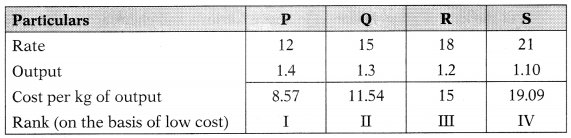Question 164.
Dec 2017: During the time of inflation, the method of pricing of material issue which leads to a lower material costs for a job is:
(A) FIFO
(B) LIFO
(C) HIFO
(D) Standard Pricing Method
(A) FIFO

Question 165.
Dec 2017: A Ltd. submits following data:
Normal uses (unit per week): 50 Minimum usage (unit per week): 25 Maximum usage (unit per week): 75 Re-order period (weeks): 4-6 Calculate re-order level.
(A) 100 units
(B) 200 units
(C) 150 units
(D) 450 units
(D) 450 units
Re-Order Level = (Maximum Usage × Maximum Delivery Period)
= (25 × 4)
= 100 units

Question 166.
Dec 2017: If the annual carrying cost of Material Z is ₹ 4 per unit and its total carrying cost is ₹ 12,000 p.a., the economic order quantity of material is:
(A) 3,000 units
(B) 4,000 units
(C) 5,000 units
(D) 6,000 units
(D) 6,000 units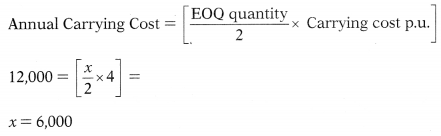Question 167.
Dec 2017: The most suitable inventory control technique for spare parts is:
(A) ABC analysis
(B) VED analysis
(C) JIT analysis
(D) Control ratios
(B) VED analysis

Question 168.
Dec 2017: Under LIFO method, the purchases and issues are as follows:
March 1 Purchased 300 units @ ₹ 3 each
March 5 Purchased 600 units @ ₹ 4 each
March 6 Issued 500 units
March 12 Purchased 700 units @₹ 5 each
March 16 Issued 800 units
The value of closing stock shall be:
(A) ₹ 900
(B) ₹ 1,200
(C) ₹ 1,500
(D) ₹ 2,100
(A) ₹ 900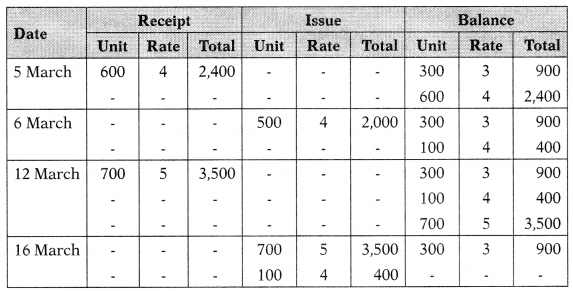Question 169.
June 2018: Which of the following is not a cost price method of pricing of material issues?
(A) First-in-first-out (FIFO)method
(B) Last-in-first-out (LIFO) method
(C) Standard price method
(D) Specified price method
(D) Specified price method

Question 170.
June 2018: Which of the following is not correct for calculation of re-ordering level of inventory?
(A) Maximum consumption X Maximum re-order period
(B) (Maximum consumption X Lead time) + Safety stock
(C) Minimum level + Consumption during time lag period
(D) (Maximum consumption X Lead time) – Safety stock
(B) (Maximum consumption X Lead time) + Safety stock

Question 171.
June 2018: ……….. is a value based system of inventory control, in which materials are analyzed according to their value so that costly and more valuable materials are given greater attention.
(A) MAX-MIN plan
(B) Review of slow and non moving items
(C) ABC Analysis
(D) Order cycling system
(C) ABC Analysis

Question 172.
June 2018: For the financial year ended 31st March, 2017, the figures extracted from the balance sheet of EXE Ltd. are as follow:
Opening stock ₹ 29,000 Closing stock ₹ 31,000 Cost of goods sold ₹ 2,40,000 The stock turnover ratio will be:
(A) 12 times
(B) 10 times
(C) 8 times
(D) 9 times
(C) 8 times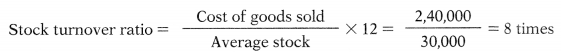Question 173.
June 2018: If EOQ is 200 units, ordering cost is ₹ 20 per order and total purchases is 4,000 units. The carrying cost per unit will be:
(A) ₹ 4
(B) ₹ 6
(C) ₹ 8
(D) ₹ 2
(A) ₹ 4
$$\mathrm{EOQ}=\sqrt{\frac{2 \times \text { Annual consumption } \times \text { Ordering cost }}{\text { Carrying cost p. u. p. a }}}$$
Put the figures given in option in above formula and check at which figure EOQ
comes 200.
Option A is correct.

Question 174.
June 2018: Which of the following items can be classified as “C” as per ABC analysis of inventory control?
Items Annual usage Unit Value per Unit (7)(A) Item number 5 only
(B) Item number 2 only
(C) Item numbers 3 and 4
(D) Item numbers 1 and 2
(A) Item number 5 onlyQuestion 175.
Dec 2018: In ……….. technique of inventory control, quantities in hand of each item or class of stock is reviewed periodically say 30, 45 or 60 days.
(A) ABC Analysis
(B) Two-bin System
(C) Order Cycling System
(D) Perpetual Inventory System
(C) Order Cycling System

Question 176.
Dec 2018: If the Minimum Stock Level is 2,500 units, Normal Consumption is 150 units, Maximum Re-order Period is 10 days and Normal Re-order Period is 8 days, then Re-order Level will be:
(A) 1,500 units
(B) 4,000 units
(C) 1,200 units
(D) 3,700 units
(D) 3,700 units

Question 177.
Dec 2018: If annual total carrying cost, per unit carrying cost and cost per order are ₹ 15,000, ₹ 10 ₹ 150 respectively, then Economic Order Quantity will be:
(A) 1,500 units
(B) 3,000 units
(C) 100 units
(D) 200 units
(D) 200 units

Question 178.
Dec 2018: Which of the following documents records quantity and value of the material?
(A) Bin card
(B) Stores ledger
(C) Both (A) and (B)
(D) None of (A) and (B)
(B) Stores ledger

Question 179.
…………. method of pricing of material issues is not popular as it always undervalues the stock and leads to creation of secret reserve.
(A) Weighted Average Price
(B) Base Stock
(C) Highest in First Out
(D) Standard Price
(C) Highest in First Out

Question 180.
Dec 2018: The monthly requirement of a component is 4,000 units. The cost per order is ₹ 1,000 and the carrying cost per unit per annum is ₹ 24. The Economic Ordering Quantity is:
(A) 2,000 units
(B) 4,000 units
(C) 577.35 units
(D) 1,825.74 units
(A) 2,000 units

Question 181.
Dec 2018: During the time of inflation, which method of pricing of material issues leads to a higher material costs for a job?
(A) First in first out method
(B) Last in first out method
(C) Highest in first out method
(D) Standard pricing method
(B) Last in first out method

Question 182.
June 2019: The following information is given:
10,000 units of material are consumed per year; per unit cost is Answer: 20; cost of processing an order is ₹ 50; Annual interest rate is 5%; Annual carrying cost of material per unit is 15% (other than interest). What would be the Economic Order Quantity (EOQ)?
(A) 200 units
(B) 500 units
(C) 400 units
(D) 100 units
(B) 500 units

Question 183.
June 2019: Which of the following is the objective of inventory management?
(A) To ensure timely delivery of inventory for production
(B) To avoid under or over production
(C) To maintain investment in inventories at lowest level
(D) All of the above
(D) All of the above

Question 184.
June 2019: V Ltd. is the manufacturer of picture tubes for TV, The following are details of their operation. Minimum usages 50 tubes per week, Maximum usages 200 tubes per week; Normal usages 100 tubes per week; lead time to supply 4-6 weeks; and Re-order quantity 400 tubes. What would be the maximum and minimum level of stock?
(A) 1,400 units and 700 units
(B) 1,200 units and 700 units
(C) 1,300 units and 600 units
(D) 1,100 units and 600 units
(A) 1,400 units and 700 units

Question 185.
June 2019: Smoke, dust, gases and loss of weight due to seasoning are examples of
(A) Scrap
(B) Spoilage
(C) Defectives
(D) Waste
(D) Waste

Question 186.
June 2019: The following information is given for receipts and issues of a material in the month of March, 2019.